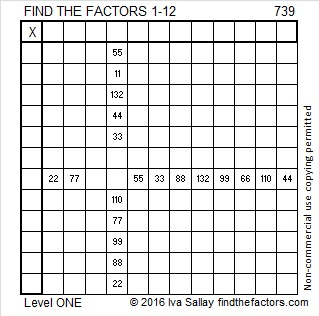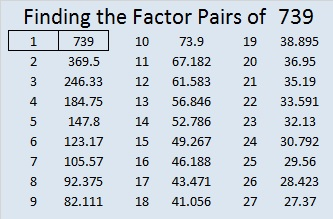# 739 and Level 1

Contents

### Today’s Puzzle:Print the puzzles or type the solution on this excel file: 12 Factors 2016-01-11

### Factors of 739:

• 739 is a prime number.
• Prime factorization: 739 is prime.
• The exponent of prime number 739 is 1. Adding 1 to that exponent we get (1 + 1) = 2. Therefore 739 has exactly 2 factors.
• Factors of 739: 1, 739
• Factor pairs: 739 = 1 x 739
• 739 has no square factors that allow its square root to be simplified. √739 ≈ 27.1845544.How do we know that 739 is a prime number? If 739 were not a prime number, then it would be divisible by at least one prime number less than or equal to √739 ≈ 27.2. Since 739 cannot be divided evenly by 2, 3, 5, 7, 11, 13, 17, 19, or 23, we know that 739 is a prime number.

### More about the Number 739:

739 is prime so there is only one way it can be written as the sum of consecutive numbers:

• 369 + 370 = 739.

However, 739 can be written as the sum of 3 squares 3 different ways:

• 27² + 3² + 1² = 739
• 21² + 17² + 3² = 739
• 17² + 15² + 15² = 739

739 is included in this list of prime numbers.

### Factors for Today’s Puzzle:This site uses Akismet to reduce spam. Learn how your comment data is processed.